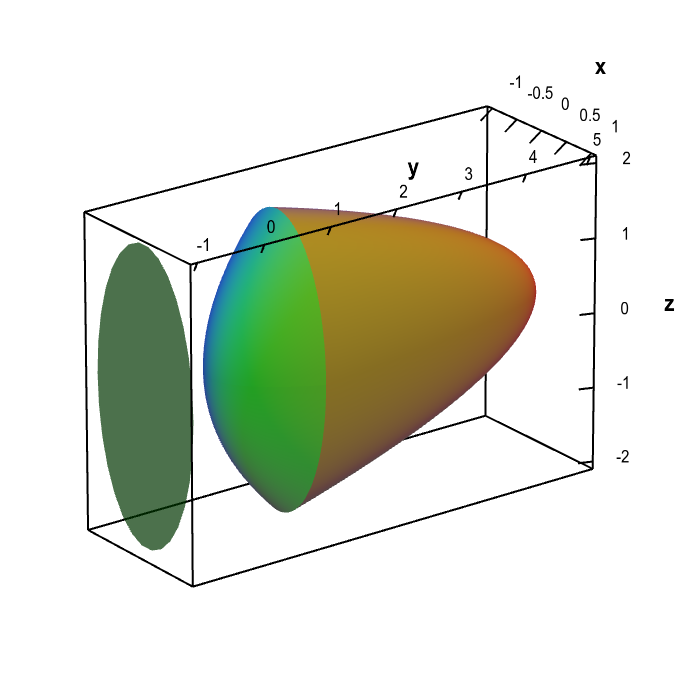# Math Insight

### Applet: Region bounded by paraboloids demonstrating the shadow methodThe region $\dlv$ is bounded by the elliptic paraboloids $y=5-4 x^2-z^2$ and $y=x^2+z^2/4$. If the sun is viewed to be in the direction of the positive $y$-axis, the shadow of $\dlv$ in a plane perpendicular to the $y$-axis is the region inside the ellipse $x^2+z^2/4=1$.